﻿

3 methods to treat outliers in machine learning

# 3 methods to treat outliers in machine learningOutliers are data points that is distant from the rest. They may be due to variability in the measurement or may indicate experimental errors.

If possible, outliers should be excluded from the data set. However, detecting that anomalous instances might be difficult, and is not always possible.

Neural Designer contains all these methods so that you can apply them in practice. You can download a free trial here.

## Introduction

Machine learning algorithms are susceptible to the statistics and distribution of the input variables. Data outliers can spoil and mislead the training process. That results in longer training times, less accurate models, and poor results.

In this post, we introduce three different methods of dealing with outliers:

1. Univariate method: This method looks for data points with extreme values on one variable.
2. Multivariate method: Here, we look for unusual combinations of all the variables.
3. Minkowski error: This method reduces the contribution of potential outliers in the training process.

To illustrate those methods, we generate a data set from the following function

$$y = sin{(pi x)}$$

Then, we replace two (y) values for those far from our function. The following chart depicts this data set.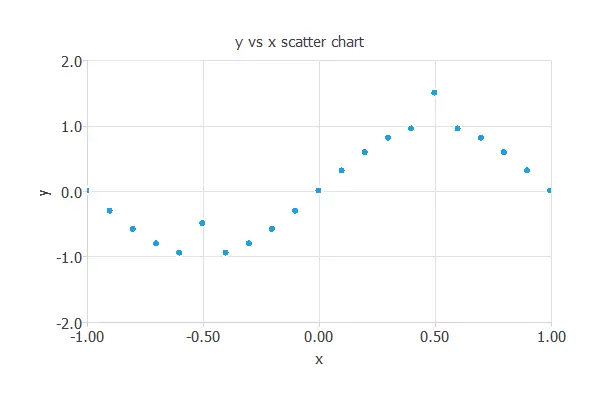The points (A=(-0.5,-1.5)) and (B=(0.5,0.5)) are outliers. Point (A) is outside the range defined by the (y) data, while Point (B) is inside that range. As we will see, that makes them of a different nature, and we will need different methods to detect and treat them.

## 1. Univariate method

One of the simplest methods for detecting outliers is the use of box plots. A box plot is a graphical display for describing the distributions of the data. Box plots use the median and the lower and upper quartiles.

Tukey’s method defines an outlier as those values of a variable that fall far from the central point, the median.

The cleaning parameter is the maximum distance to the median that will be allowed. The test becomes less sensitive to outliers if the cleaning parameter is large. On the contrary, many values are detected as outliers if it is too small.

The following chart shows the box plot for the variable (y).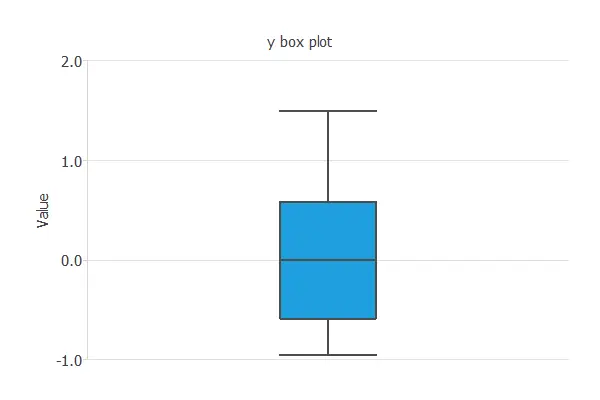As we can see, the minimum is far away from the first quartile and the median. If we set the cleaning parameter to 0.6, Tukey’s method detects Point (A) as an outlier and cleans it from the data set.

Plotting the box plot for that variable again, we can notice that the outlier has been removed. As a consequence, the distribution of the data is now much better.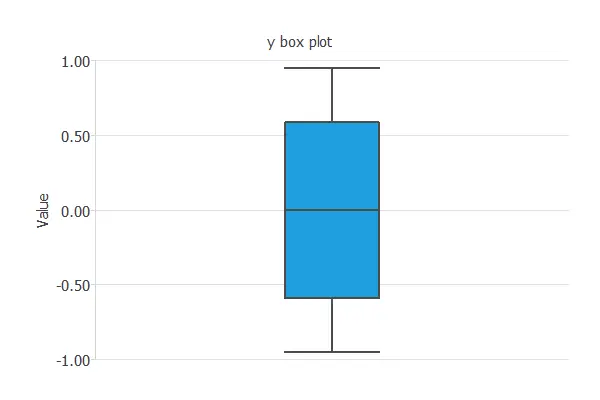However, this univariate method has not detected Point (B), so we are not finished.

## 2. Multivariate method

Outliers do not need to be extreme values. Indeed, as we have seen with Point (B), the univariate method does not always work well. The multivariate method tries to solve that by building a predictive model using all the data available and cleaning those instances with errors above a given value.

In this case, we have trained a neural network using all the available data (but Point (A), excluded by the univariate method). Then, we perform a linear regression analysis to obtain the next graph. The predicted values are plotted versus the real ones. The coloured line indicates the best linear fit, and the grey line indicates a perfect fit.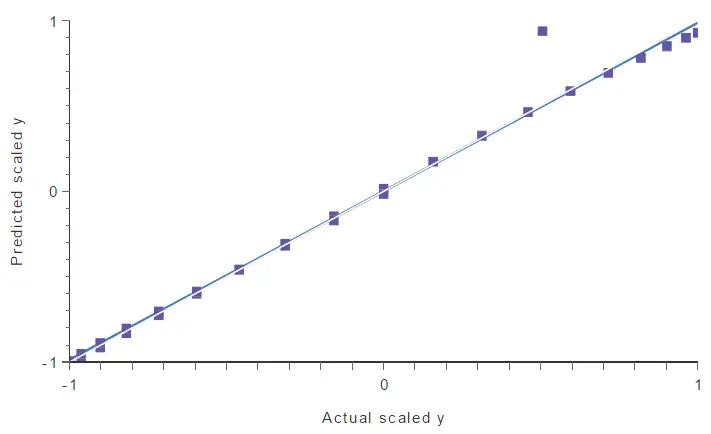In the above chart, a point falls too far from the model. This point spoils the model, and therefore it might be another outlier.

To find that point quantitatively, we can calculate the maximum errors between the outputs from the model and the targets. The following table lists the five instances with maximum errors.

Instance Error X Y
11 0.430 0.500 0.500
10 0.069 0.550 0.987
12 0.067 0.450 0.987
9 0.064 0.600 0.951
13 0.058 0.400 0.951

As we can notice, sample 11 has a significant error compared to the others. If we look at the linear regression chart, we see that this instance matches the point far from the model.

By selecting 20% of maximum error, this method identifies Point B as an outlier and cleans it from the data set. We can see that by performing a linear regression analysis again.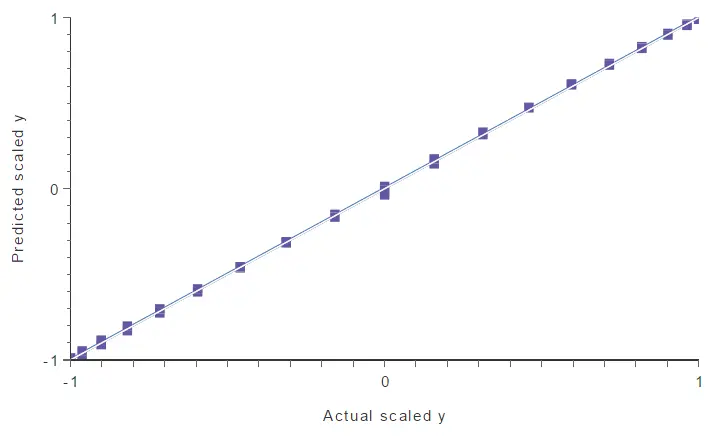There are no more outliers in the data set, so the neural network’s generalization capabilities improve notably.

## 3. Minkowski error

Now, we talk about a different method for dealing with outliers. Unlike the univariate and multivariate methods, it doesn’t detect and clean the outliers. Instead, it reduces the impact that outliers will have on the model.

The Minkowski error is a loss index that is more insensitive to outliers than the standard mean squared error.

The mean squared error raises each instance error to the square, making a too big contribution of outliers to the total error,

$$mean_squared_error = frac{sum left(outputs – targetsright)^2}{instances_number}$$

The Minkowski error solves that by raising each instance error to a number smaller than 2. This number is called the Minkowski parameter, and reduces the contribution of outliers to the total error,

$$minkowski_error = frac{sumleft(outputs – targetsright)^{minkowski_parameter}}{instances_number}$$

A typical value for the Minkowski parameter is 1.5.

For instance, if an outlier has an error of 10, the squared error for that instance is (10^2=100), while the Minkowski error is (10^{1.5}=31.62).

To illustrate this method, we build two different neural networks from our data set, containing two outliers ((A) and (B)). The architecture selected for this network is 1:24:1. The loss index for the first neural network is the mean squared error. The loss index for the second neural network is the Minkowski error.

The neural network trained with the mean squared error is plotted in the following figure. As we can see, two outliers are spoiling the model.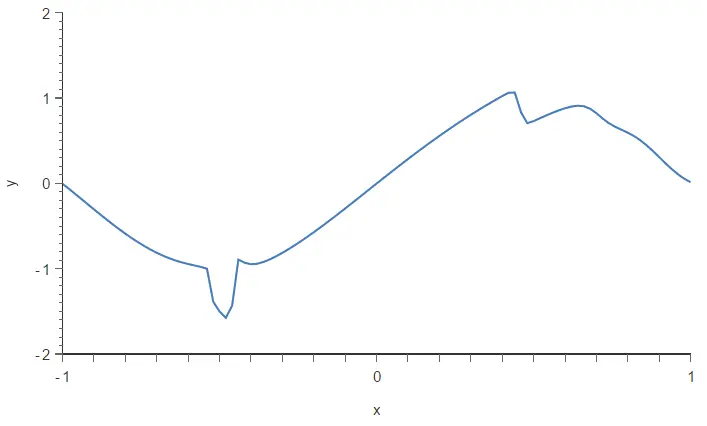Now, we train the same neural network with the Minkowski error. The following figure depicts the resulting model.As a result, the Minkowski error has made the training process more insensitive to outliers and has improved our model’s quality.

## Conclusions

We have seen that outliers are one of the main problems when building a predictive model. Indeed, they cause data scientists to achieve more unsatisfactory results than they could. We need practical methods to deal with that spurious points and remove them to solve that.

In this article, we have seen 3 different methods for dealing with outliers: the univariate method, the multivariate method, and the Minkowski error. These methods are complementary, and we might need to try them all if our data set has many severe outliers.